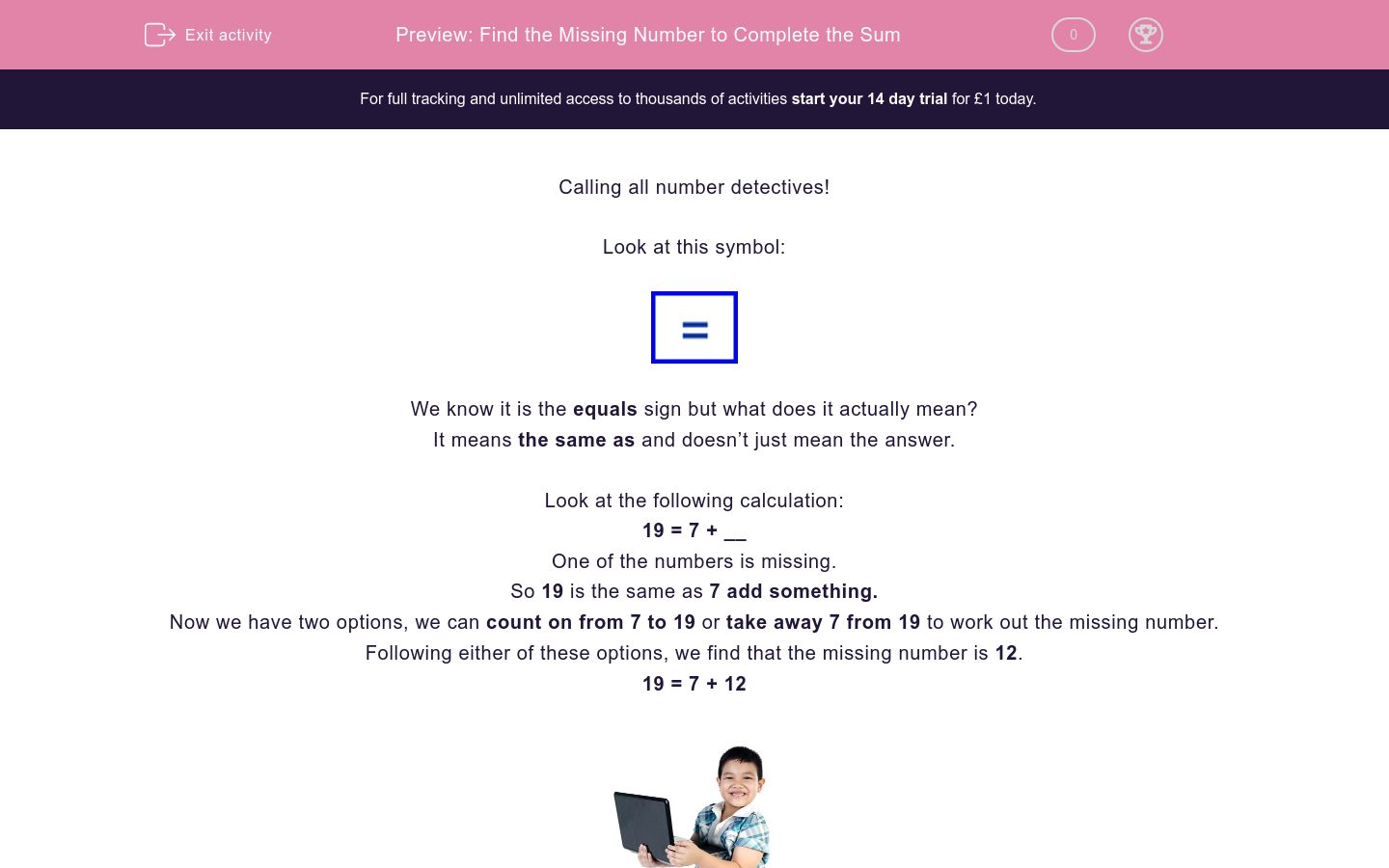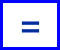# Find the Missing Number to Complete the Sum

In this worksheet, students will complete a calculation by adding in the missing numbers. It will strengthen their number skills.Key stage:  KS 2

Curriculum topic:   Verbal Reasoning

Curriculum subtopic:   Complete the Calculation

Difficulty level:### QUESTION 1 of 10

Calling all number detectives!

Look at this symbol:We know it is the equals sign but what does it actually mean?

It means the same as and doesn’t just mean the answer.

Look at the following calculation:

19 = 7 + __

One of the numbers is missing.

So 19 is the same as 7 add something.

Now we have two options, we can count on from 7 to 19 or take away 7 from 19 to work out the missing number.

Following either of these options, we find that the missing number is 12.

19 = 7 + 12Let’s try another:

17 = 20 - __

In this question, the answer of 17 is the same as 20 take away something.

What do we need to take away from 20 to reach 17?

The missing number is 3.

17 = 20 - 3

Remember that equals means the same as and the answers on either side of the equals sign must be the same.

In this activity, you will be completing sums by finding the missing number.

Good luck!

Welcome to another lovely activity, number detective. Let's get stuck in straight away!

Below is a calculation. One of the numbers has been taken away by the cheeky number burglar! Can you work out what it is ?

__ x 3 = 21Are you ready for another one, number detective?

Can you work out what number is missing from the calculation below

__ - 50 = 62Here we go again ...!

Below is another calculation that is missing a number. Can you work out what number has been left out, number detective?

__ - 23 = 80Ready for another one, number detective?

Can you work out what number is missing from the calculation below?

3 x __ = 36.Surely we're not halfway through already, number detective?

Can you work out what number is missing from the calculation below?

__ + 26 = 107Take a look at the calculation below. Can you work out what the missing number is?

109 - __ = 70Here's another calculation which is missing a number. Can you work out what the missing number is, number detective?

__ + 25 = 117You're nearly there, number detective - don't give up now!

Can you work out what number is missing from the calculation below?

3 x __ = 24Only two more questions to go!

I think you know what to do now!

Can you work out the missing number in the calculation below?

122 - __ = 80Brilliant achievement, number detective - you've reached the final activity!

So, just for a change - can you work out what number is missing from the calculation below?

124 - __ = 40• Question 1

Welcome to another lovely activity, number detective. Let's get stuck in straight away!

Below is a calculation. One of the numbers has been taken away by the cheeky number burglar! Can you work out what it is ?

__ x 3 = 217
EDDIE SAYS
How did you get on? The best way to solve this puzzle is to use the opposite calculation to the one in the question - that means division because that is the opposite of multiplication. For this question, therefore, you need to find out how many threes there are in 21: so, 21 divided by 3 = 7.
• Question 2

Are you ready for another one, number detective?

Can you work out what number is missing from the calculation below

__ - 50 = 62112
EDDIE SAYS
OK, let's look at this one. The symbol in this question shows that it is a subtraction sum but to find this missing number, you need to do the opposite - that is addition! So, we need to add 50 to 62 and the quickest way to do that is in five jumps of ten! Super!
• Question 3

Here we go again ...!

Below is another calculation that is missing a number. Can you work out what number has been left out, number detective?

__ - 23 = 80103
EDDIE SAYS
Have you got the idea now? To find the answer, we need to do the opposite calculation to the one in the question - that is addition because the question is subtraction. So, we need to work out: 80 + 23. This can be worked out as 80 + 10 + 10 + 3 = 103. Brilliant!
• Question 4

Ready for another one, number detective?

Can you work out what number is missing from the calculation below?

3 x __ = 36.12
EDDIE SAYS
Are you getting more confident with these yet? Keep on practising and you'll find the whole thing becomes much easier. In this question, you need to do the opposite of multiplication and work out how many threes there are in 36: 36 divided by 3 = 12.
• Question 5

Surely we're not halfway through already, number detective?

Can you work out what number is missing from the calculation below?

__ + 26 = 10781
EDDIE SAYS
This time, you need to take away 26 from 107. This can be worked out as 107 - 10 - 10 - 6 = 81. Remember that when you're subtracting blocks of tens from a number, the units column in that number will stay the same.
• Question 6

Take a look at the calculation below. Can you work out what the missing number is?

109 - __ = 7039
EDDIE SAYS
You're doing great! You could work this one out by simply counting on from 70 to 109 in blocks of ten: 70 + 10 + 10 + !0 = 100 and then adding the units: 100 + 9 = 109. You've added on 39. Alternatively, you could subtract 70 from 109 in seven blocks of ten to get the answer of 39. You choose which method works best for you.
• Question 7

Here's another calculation which is missing a number. Can you work out what the missing number is, number detective?

__ + 25 = 11792
EDDIE SAYS
This calculation can be worked out by using the opposite operation to the one that has been given. So, the opposite operation to adding is subtracting: 117 - 25 can be worked out as 117 - 10 - 10 - 5 = 92.
• Question 8

You're nearly there, number detective - don't give up now!

Can you work out what number is missing from the calculation below?

3 x __ = 248
EDDIE SAYS
Did you find the missing number, Detective? Here you need to do the opposite of multiplication, which is division. You need to find how many threes are in 24. 24 divided by 3 = 8.
• Question 9

Only two more questions to go!

I think you know what to do now!

Can you work out the missing number in the calculation below?

122 - __ = 8042
EDDIE SAYS
You're becoming a number expert! The method here is to subtract 80 from 122. So you can make eight backwards jumps of ten. Simple really isn't it?!
• Question 10

Brilliant achievement, number detective - you've reached the final activity!

So, just for a change - can you work out what number is missing from the calculation below?

124 - __ = 4084
EDDIE SAYS
To find the answer to this final question you need to subtract 40 (or four backwards jumps of 10) from 124. This activity is now all done, I hope you enjoyed the challenge, number detective!
---- OR ----

Sign up for a £1 trial so you can track and measure your child's progress on this activity.

### What is EdPlace?

We're your National Curriculum aligned online education content provider helping each child succeed in English, maths and science from year 1 to GCSE. With an EdPlace account you’ll be able to track and measure progress, helping each child achieve their best. We build confidence and attainment by personalising each child’s learning at a level that suits them.

Get started